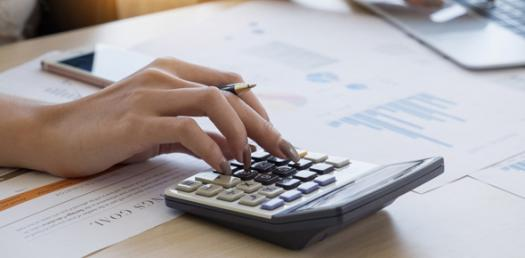# The Ultimate Business Math Test Quiz #4

20 Questions | Total Attempts: 140SettingsCreate your own Quiz.

• 1.
Which of the following is the correct fraction of 5.6 %
• A.

7/125

• B.

28/50

• C.

28/500

• D.

A & C

• 2.
What is the decimal of 58 2/3 percentage
• A.

0.5867

• B.

0.5844

• C.

58.67

• D.

58.44

• 3.
Which of the following is the percentage of 1.5
• A.

50%

• B.

75%

• C.

100%

• D.

125%

• E.

None

• 4.
Which of the following is percentage of 3 14/15
• A.

3.56%

• B.

356%

• C.

0.356%

• D.

None

• 5.
Labour got one-twenth of increase in his wages What percentage of increase he got in his wages
• A.

1.20%

• B.

0.2%

• C.

20%

• D.

120%

• 6.
18% of 120,000
• A.

20,600

• B.

18,000

• C.

21,600

• D.

None

• 7.
32% of 850 grams
• A.

320 grams

• B.

250 grams

• C.

262 grams

• D.

272 grams

• 8.
What quantity has its 30% is equal to 15
• A.

4.5

• B.

45

• C.

50

• D.

40

• 9.
A university has 1500 students. 40% of students belong from district Mirpur Khas, how many students belong from other than Mirpurkhas?
• A.

600

• B.

900

• C.

500

• D.

100

• 10.
Mr. Ahmed earns 75,000 per month, his saving and expense ratio is 1:3. i. calculate the saving amount and ii. percentage of saving
• A.

I. 20,000, ii. 20%

• B.

I. 56,250, ii. 75%

• C.

I. 18,750, ii. 25%

• D.

I. 18750, ii. 75%

• 11.
A batsman scored 120 runs which included 4 boundaries and 7 sixes. What percent of his total score did he make by running between the wickets?
• A.

51.67%

• B.

48.33%

• C.

62%

• D.

58%

• 12.
Two students appeared at an examination. One of them secured 9 marks more than the other and his marks was 56% of the sum of their marks. The marks obtained by them are:
• A.

39, 30

• B.

41, 32

• C.

42, 33

• D.

43, 34

• 13.
A vegetable seller had some okra. He sells 30% of okra and still has 5 kg. Originally, he had:
• A.

7 KG

• B.

7.14 KG

• C.

6.5 KG

• D.

8.5 KG

• 14.
In an election between two candidates, one got 55% of the total valid votes, 20% of the votes were invalid. If the total number of votes was 7500, the number of valid votes that the other candidate got, was:
• A.

2700

• B.

2900

• C.

3000

• D.

3100

• E.

None

• 15.
The price of a house is decreased from Rupees Fifteen lakhs to Rupees Twelve lakhs. Find the percentage of decrease.
• A.

15%

• B.

20%

• C.

25%

• D.

30%

• 16.
Asghar buys a colour T.V set for Rs. 12,200 and sells it at a loss of 20%. What is the selling price of the T.V set?
• A.

9,760

• B.

2,440

• C.

10500

• D.

12,160

• 17.
ABC company earns revenue 500,000 and suffers a 12% loss. Calculate the total expense of ABC company
• A.

520,000

• B.

540,000

• C.

560,000

• D.

580,000

• 18.
Rs. 5000 suit is on sale for 25% off. How much is the suit after the discount?
• A.

4000

• B.

3750

• C.

3500

• D.

3300

• 19.
A buyer purchased a gift for Rs 550. The profit margin of the seller was 10%. Before reaching home buyer changed his idea and exchange the same gift with different colors and paid an extra amount of Rs. 50 for the exchange. Find the seller's profit and profit percent.
• A.

10%

• B.

15%

• C.

20%

• D.

25%

• 20.
The marked price of a cement bag is Rs. 300. if a discount of 10% is allowed then what would be its sale price?
• A.

330

• B.

Rs. 290

• C.

Rs. 270

• D.

Rs. 280

Related TopicsBack to top
×

Wait!
Here's an interesting quiz for you.